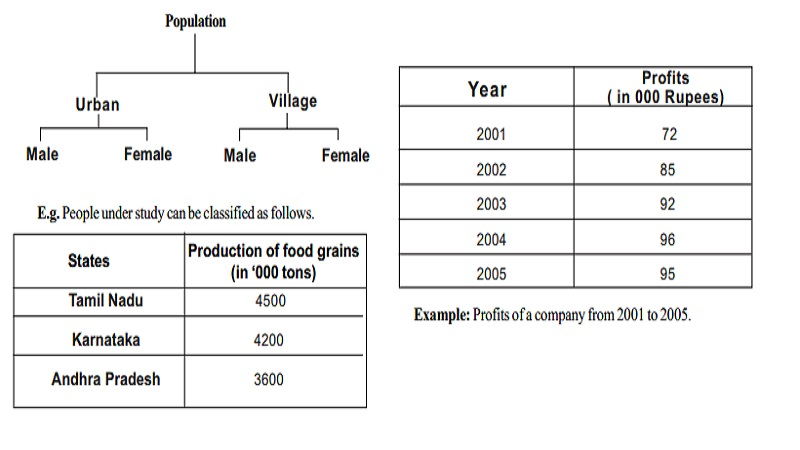Home | | Economics | | Economics | Statistical Analysis : Classification of Data

# Statistical Analysis : Classification of DataThere are four types of classification. They are Geographical classification, Chronological classification, Qualitative classification, Quantitative classification.

## Classification of Data

Classification is the process of arranging the collected data into classes and to subclasses according to their common characteristics. Classification is the grouping of related facts into classes. E.g. sorting of letters in post office

## Types of classification

There are four types of classification. They are

Geographical classification

Chronological classification

Qualitative classification

Quantitative classification

## (i) Geographical classification

When data are classified on the basis of location or areas, it is called geographical classification

Example: Classification of production of food grains in different states in India.

States          Production of food grains

(in '000 tons)

Karnataka   4200

## (ii) Chronological classification

Chronological classification means classification on the basis of time, like months, years etc.

Year  Profits

( in 000 Rupees)

2001  72

2002  85

2003  92

2004  96

2005  95

Example: Profits of a company from 2001 to 2005.

Profits of a company from 2001 to 2005

## (iii) Qualitative classification

In Qualitative classification, data are classified on the basis of some attributes or quality such as sex, colour of hair, literacy and religion. In this type of classification, the attribute under study cannot be measured. It can only be found out whether it is present or absent in the units of study.

## (iv) Quantitative classification

Quantitative classification refers to the classification of data according to some characteristics, which can be measured such as height, weight, income, profits etc.

Example: The students of a school may be classified according to the weight as follows

Weight (in kgs)    No of Studemts

40-50 50

50-60 200

60-70 300

70-80 100

80-90 30

90-100        20

Total        700

There are two types of quantitative classification of data. They are

Discrete frequency distribution

Continuous frequency distribution

In this type of classification there are two elements (i) variable (ii) frequency

Variable

Variable refers to the characteristic that varies in magnitude or quantity. E.g. weight of the students. A variable may be discrete or continuous.

Discrete variable

A discrete variable can take only certain specific values that are whole numbers (integers). E.g. Number of children in a family or Number of class rooms in a school.

Continuous variable

A Continuous variable can take any numerical value within a specific interval.

Example: the average weight of a particular class student is between 60 and 80 kgs.

Frequency

Frequency refers to the number of times each variable gets repeated.

For example there are 50 students having weight of 60 kgs. Here 50 students is the frequency.

Frequency distribution

Frequency distribution refers to data classified on the basis of some variable that can be measured such as prices, weight, height, wages etc.

The following are the two examples of discrete and continuous frequency distribution

The following technical terms are important when a continuous frequency distribution is formed

Class limits: Class limits are the lowest and highest values that can be included in a class. For example take the class 40-50. The lowest value of the class is 40 and the highest value is 50. In this class there can be no value lesser than 40 or more than 50. 40 is the lower class limit and 50 is the upper class limit.

Class interval: The difference between the upper and lower limit of a class is known as class interval of that class. Example in the class 40-50 the class interval is 10 (i.e. 50 minus 40).

Class frequency: The number of observations corresponding to a particular class is known as the frequency of that class

Example:

Income (Rs)         No. of persons

1000 - 2000         50

In the above example, 50 is the class frequency. This means that 50 persons earn an income between Rs.1, 000 and Rs.2, 000.

(iv) Class mid-point: Mid point of a class is formed out as follows.

Tabulation of Data

A table is a systematic arrangement of statistical data in columns and rows. Rows are horizontal arrangements whereas the columns are vertical ones.

Study Material, Lecturing Notes, Assignment, Reference, Wiki description explanation, brief detail

Related Topics